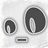# Return Offer工资哪家强？脸书亚麻微软领英丢盒子逐个数！

8月底了，新学期要开始了，在美国各公司里暑假实习的各位，是不是也在收拾行囊，正在或者已经返校了呢？123000*1.1+220000/4+75000/4 = 209050

FB今年sign on的上限依然是10万美元，所以硕士生的顶配return offer是这样的：123000*1.1+220000/4+100000/4 = 215300150000*1.1+320000/4+75000/4 = 263750

It seems 160k, 300k, 75k is the most common package.

160000*1.1+300000/4+75000/4 = 269750

PhD对应着E4，级别比Master（E3）高一级，工资上限也更高：160000*1.1+400000/4+75000/4 = 294750

160000*1.1+400000/4+75000/4 = 301000150000*1.1+150000/4+40000/4 = 212500UX的级别也是IC，上图是IC4的return offer：

130000*1.1+70000/4+70000/4 = 178000level 60

118000*1.2+130000/4+5000 ~ 225000 = 179100 ~ 199100110000*1.1+150000/4+50000/4 = 171000135000*1.1+200000/4+30000/4 = 206000130000*1.05+160000/4+35000/4 = 185250128000+95000*0.05+40000 = 172750